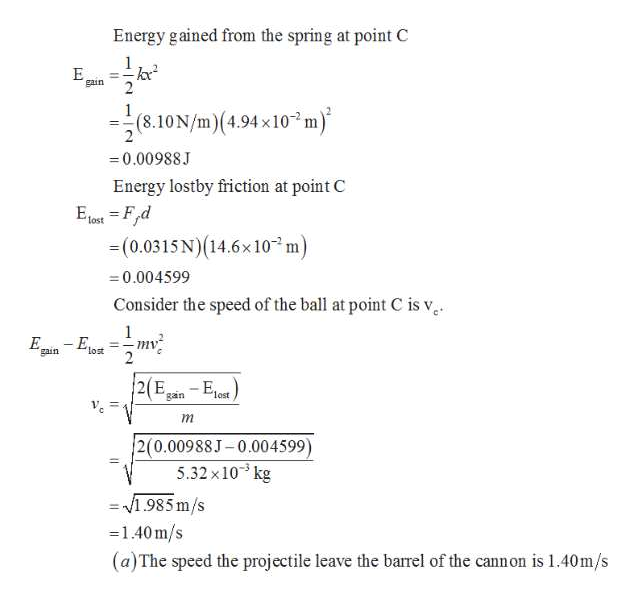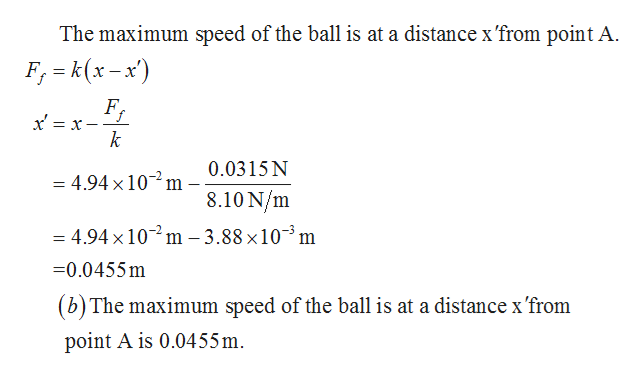# A toy cannon uses a spring to project a 5.32-g soft rubber ball. The spring is originally compressed by 4.94 cm and has a force constant of 8.10 N/m. When the cannon is fired, the ball moves 14.6 cm through the horizontal barrel of the cannon, and the barrel exerts a constant friction force of 0.0315 N on the ball.(a) With what speed does the projectile leave the barrel of the cannon?(b) At what point does the ball have maximum speed?(c) What is this maximum speed?

Question

A toy cannon uses a spring to project a 5.32-g soft rubber ball. The spring is originally compressed by 4.94 cm and has a force constant of 8.10 N/m. When the cannon is fired, the ball moves 14.6 cm through the horizontal barrel of the cannon, and the barrel exerts a constant friction force of 0.0315 N on the ball.

(a) With what speed does the projectile leave the barrel of the cannon?

(b) At what point does the ball have maximum speed?

(c) What is this maximum speed?

check_circleExpert Solution
Step 1

The ball moves from point A and leaves the barrel of the cannon at point C is shown below .

Step 2

Given that the mass of the ball is m=5.32×10-3kg, force constant of the spring is k=8.10N/m, and the spring is compressed by 4.94m.help_outlineImage TranscriptioncloseEnergy gained from the spring at point c 1 gain 1 (8.10N/m)(4,94 x10 m) 2 -0.00988J Energy lostby friction at point C E locet =Fd (0.0315 N) (14.6x10 m) tost 0.004599 Consider the speed of the ball at point C is v 1 mv lost gain lost gain m 2(0.00988J-0.004599) 5.32x10 kg =1985 m/s -1.40m/s (a)The speed the projectile leave the barrel of the cannon is 1.40m/s fullscreen
Step 3

Continuing previ...help_outlineImage TranscriptioncloseThe maximum speed of the ball is at a distance x'from point A. F, k(x-x F k 0.0315 N 4.94 x 102 m 8.10 N/m =4.94 x10m-3.88x103m -0.0455m (b) The maximum speed of the ball is at a distance x 'from point A is 0.0455m fullscreen

### Want to see the full answer?

See Solution

#### Want to see this answer and more?

Solutions are written by subject experts who are available 24/7. Questions are typically answered within 1 hour*

See Solution
*Response times may vary by subject and question
Tagged in

### Work,Power and Energy